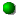# \$B%Q%s%9%?!<%:WB@1(B = \$B%U%k%9WB@1(B

P/2013 J4 ( PanSTARRS ) = P/2019 Y2 ( Fuls )###\$B2s5"5-O?(B\$BH/8+(B\$B=P8=(B\$B4QB,\$5\$l\$J\$+\$C\$?2s5"(B\$BH/8+A0\$N=P8=(B\$BH/8+A0\$N4QB,\$5\$l\$J\$+\$C\$?2s5"(B\$B>-Mh\$N2s5"(BP/2019 Y2 2020/01/31P/2013 J4 2013/08/27##### P/2013 J4 ( PanSTARRS ) \$B\$H(B P/2019 Y2 ( Fuls ) \$B\$NF1Dj\$O!"(BSam Deen\$B;a\$K\$h\$C\$F;XE&\$5\$l!"(BCBET 4792\$B\$G8xI=\$5\$l\$?!#(B